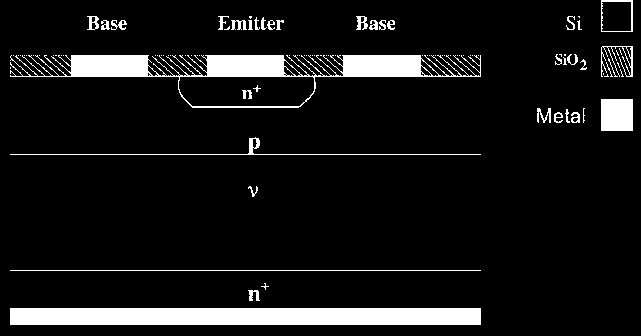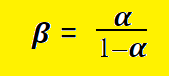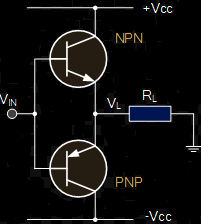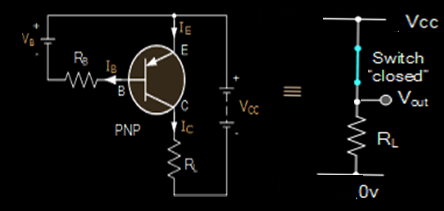# PNP Transistor Working and Application Explained

A PNP transistor is nothing but a bipolar junction transistor (BJT). It is made by sandwiching an n-type semiconductor between the two p-type semiconductors. This transistor is a three-terminal device. The terminals are namely, emitter (E), base (B), and collector (C). The PNP transistor acts as two PN junction diodes connected one after another. These diodes make junctions as are connected one after another and are called as collector-base and base-emitter junction.

In PNP transistors, the majority charge (current) carriers are holes and the electrons are the minority charge carriers. To understand these carriers, terminals, and many more we need to go through the basics of transistors. Thus, let us learn more about all this in the upcoming sections.

## Bipolar Junction Transistor

Popularly known as BJT, is a solid-state current controlled device. It is useful in switching circuits electronically. It has three terminals in which the current applied to the base region controls the current flow in emitter and collector.Bipolar Junction Transistor

Therefore, it’s like we can control the transistor action by differing the amount of applied current at the base terminal. Hence, the name current controlled device. Bipolar junction Transistor is of two types, namely, NPN and PNP. In NPN, the P-type semiconductor is sandwiched between the two N-type semiconductors. The only difference in the symbols of the two transistors is that in NPN, the current direction is from base to emitter and in PNP it’s opposite, i.e., from emitter to base.

### NPN SymbolNPN Transistor

## PNP Transistor Construction and Symbol

In PNP the two P-type regions are there on the extreme and an N-type region is in between the two. Emitter and collector are always on the two extremes. Therefore, in the case of PNP, the emitter and collector region connects to P-type semiconductor and base to that with N-type.PNP Transistor Symbol

The construction can be understood by visualizing two diodes connected one after the other. The meeting point of the two diodes (cathode) becomes the base terminal and the two anodes at the extremes become the emitter and collector of the NPN transistor.

The symbol of the PNP transistor is the same as fig. One thing to note here is the current direction in both NPN and PNP transistors. This helps a lot while solving numerical problems.

## Working of PNP Transistor

The circuit connection of the PNP transistor is as below. Here the emitter region has a positive bias voltage with respect to base and collector. On the other hand, the base has a negative voltage bias with respect to the emitter. The current direction and voltage polarity are just opposite to that of the NPN transistor.PNP Transistor Working

Now, the necessary condition for transistor operation is that the base voltage should be more negative than that of emitter voltage. Therefore, the base-emitter junction acts as a diode here. If we apply a small amount of current in the base region then a large current flows through the emitter to the collector region. As we mostly use silicon and germanium the base voltage is 0.7 V and 0.3 V respectively.

• From the figure, we can easily identify that the base region is the input and the emitter-collector region is the output of the transistor.
• Voltage is applied at the base terminal and load resistance (RL) is kept at the output. This is to limit the maximum current of the device.
• A negative voltage is applied to the base terminal. Also, a resistance (RB) is connected across the base terminal to limit the maximum current through the base terminal.
• If we apply Kirchoff Current Law (KCL) in the transistor circuit then, the collector current is always equal to the subtraction of the base from the emitter current,i.e., IC = IE – IB
• One more important factor here is the current gain factor, 𝜷.

### Basic Formulae of PNP Transistor

• IC = IE – IB.
• Output current / Input Current = DC Current Gain

Therefore, 𝜷 = (IC / IB)

• Combining the above two formulas, we can derive the following relations:

IC = (𝜷 * IB

IB =  (IC / 𝜷 )

For the common base transistor, current gain equals the ratio of collector current to that of the emitter current.

Therefore, 𝜶 = ( IC / IE )

Relation Between  𝜶 & 𝜷 is like:#### Output Current Equation

We can write the collector current of the PNP transistor as

IC = – 𝜶 * IE + ICBO

where,  ICBO = saturation current

Also,  IE = -( IC + IB )

IC = – 𝜶 (-(IC + IB )) + ICBO

IC – 𝜶IC =  – 𝜶IB + ICBOTherefore, from the relation between 𝜶 & 𝜷, we have

IC = 𝜷IB + (1+ 𝜷) ICBO

## Numerical Example On PNP (BJT Transistor)

Let us consider a PNP transistor which is a part of some electronic circuit. The given parameters are the base voltage, emitter voltage, and the supply voltage. For instance, take the values as 2.5V, 3V, and +10V respectively. Also, the base resistor is there having a value of 100Kohm and load resistance = emitter resistance = 2.5 Kohm. Find the values of current gain (alpha and beta) for the given transistor

So, take it as a task. Use the above formulas and try calculating the required parameters and do comment below. This is a common kind of question that one gets in the exam.

## Transistor Identification of PNP

Now, after learning the working principle and other basics of the PNP transistors, it is important to be clear to find out the right configuration whenever a bipolar junction transistor is provided. Of course, there are some differences between the structure of the two BJTs but other than that also we should note a few important points.PNP Transistor Identification

PNP is ON when a negative voltage appears at the base voltage. When a positive voltage is present at the base then it gets OFF. Other than this we have a few more tests where we calculate resistance values. Each pair of junctions (terminals) are to be tested for resistance in both directions. Let us see their operations below:

• Emitter-Collector Terminals: This region seems like a diode but it does not conduct in either direction.
• Emitter-Base Terminals: This region acts as a diode and conducts only in one direction.
• Collector-Base Terminals: This is also the same as the emitter-base terminal. Acts like a diode but conducts only in one direction.

Now, let us have a look at the resistance value for identifying PNP.

• Collector-Emitter – High Resistance
• Collector-Base      – Low Resistance
• Emitter-Collector – High Resistance
• Emitter-Base         – Low Resistance
• Base-Collector      – High Resistance
• Base-Emitter         – High Resistance

Another, very interesting and important thing to know here is that which transistor is better and why?

Let us try to find out the answer in the next section.

### PNP Versus NPN

Well, there are a few things in common. Both are bipolar junction transistor and a current controlled device. Both are used for amplification and circuit switching. The main points are as below:

• PNP stands Positive Negative Positive. NPN stands for Negative Positive Negative.
• Conduction of NPN is high and that of PNP is low. This is because in NPN conduction takes place with electrons and in PNP it takes place through the holes and but obvious the speed of the electron is more & hence the conduction.
• In NPN, we supply positive current to the base, the current flows through the collector to the emitter. Whereas, when a negative current applies at the base terminal of PNP then the current flows from emitter to collector.
• The current direction in NPN is from collector to emitter and in PNP from emitter to collector.
• Switching time is faster in NPN & slower in PNP.
• NPN turns ON when the electron enters the base region. Whereas, PNP transistor turns ON when holes enter the base terminal.
• In both the transistors, the emitter-base junction is forward biased and the collector-base junction is reverse biased.
• The ground signal is high in the case of PNP and low for NPN.
• The emitter arrow is pointed in for PNP transistor, while it is pointed out in the case of NPN transistor.

## Applications

Though most electronic circuits use NPN transistors (preferably for its speed) but still many circuits use PNP withing it. Few applications are:

• Complimentary amplifiers, like Class AB/B output stages.
• Current mirror
• Useful in driver applications where the load on one side is grounded.
• In regulators having lower dropout voltage.
• Darlington pair uses a PNP transistor.
• Useful in matched pair circuits for continuous power generation.
• Heavy motor applications for controlling current flow.
• Robotics applications.

Some other applications are discussed briefly in the next two sections.

## Transistor Matching of BJT

The first question is what this transistor matching is?

Secondly, why is it required?Transistor Matching of BJT

Well, when we connect both NPN and PNP in the single circuit then we call it transistor matching. We do this to generate more power. NPN and PNP are complementary to each other. When these are connected in amplifiers then continuous high power is generated by the transistor pair.

Now, one may ask how come this pair works continuously? Think! Think!

The answer is quite simple that the NPN conducts in the positive half cycle and the PNP conducts in the negative half cycle of the signal. Therefore, the device operates continuously (just as the full-wave rectifier). One thing to take care of is that both the transistors should have the same value of DC current gain. This pair makes a useful circuit in robotics, power amplifier applications, and motor control.

### PNP as a Switch

The above fig shows the connection for the PNP working as a switch. It is an electronic switch. For understanding, take it as a normal switch which we switch OFF and switch ON manually(for example to light up an LED). Here the same work will be done but electronically.PNP Transistor as a Switch

As the PNP transistor turns ON when a negative voltage appears at the base terminal of the transistor and gets OFF with the positive supply, therefore, this characteristic of the transistor is used to use it as a switch.

Operating modes of transistors play an important role in the applications of these transistors. Depending on the biasing conditions, three modes of operations are there. These are:

• Active Mode: In the active mode, the transistor is usually used for current amplification.
• Cutoff Mode: Both emitter-base and collector-base are reverse biased and therefore, do not allow the current to flow from collector to emitter. The current through the device is zero in this mode.
• Saturation Mode: In saturation mode, both the collector-base and the emitter-base junctions are forward biased Therefore, the current flows from collector to emitter when base-emitter voltage is high.
•
•
•
•
•
•